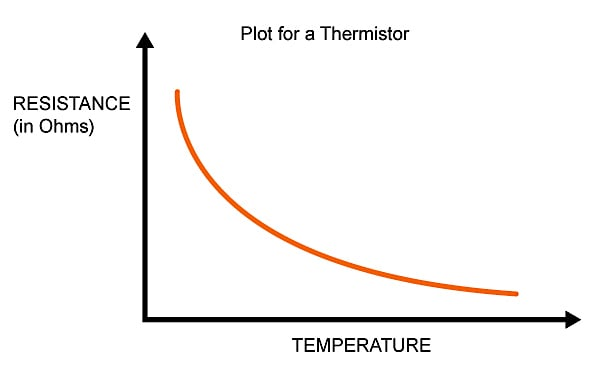﻿ 使用NTC热敏电阻测量温度的4个方法- 敏创电子

#### 电阻计算器 - 问题解答 - 网站地图 - 联系我们# 使用NTC热敏电阻测量温度的4个方法### BOM

• Arduino的
• MEGA或Uno或您最喜欢的Arduino风味
• 一些跳线
• 焊锡和烙铁（可能是因为你的热敏电阻不适合Arduino接头）

• Arduino IDE

### 理论### 找到曲线拟合公式的四种方法

#### 方法四：

1

Ť

lnC.

3

1
$\frac{}{T}=A+B\mathrm{ln}\left(R\right)+C\left(\mathrm{ln}\left(R\right){\right)}^{3}$

$\frac{1}{}$$\frac{}{T}=\frac{1}{{T}_{o}}+\left(\frac{1}{\beta }\right)\cdot \mathrm{ln}\left(\frac{R}{{R}_{o}}\right)$